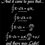# Is Summation a perfect square?

$\sum_{i=1}^{n^2} (i) = a^2$

$(n,a)\in N$ , $N$ denotes natural number.

Does there exist only one such $a$ to satisfy the above conditions?Note by Akash Shukla
5 years ago

This discussion board is a place to discuss our Daily Challenges and the math and science related to those challenges. Explanations are more than just a solution — they should explain the steps and thinking strategies that you used to obtain the solution. Comments should further the discussion of math and science.

When posting on Brilliant:

• Use the emojis to react to an explanation, whether you're congratulating a job well done , or just really confused .
• Ask specific questions about the challenge or the steps in somebody's explanation. Well-posed questions can add a lot to the discussion, but posting "I don't understand!" doesn't help anyone.
• Try to contribute something new to the discussion, whether it is an extension, generalization or other idea related to the challenge.
• Stay on topic — we're all here to learn more about math and science, not to hear about your favorite get-rich-quick scheme or current world events.

MarkdownAppears as
*italics* or _italics_ italics
**bold** or __bold__ bold
- bulleted- list
• bulleted
• list
1. numbered2. list
1. numbered
2. list
Note: you must add a full line of space before and after lists for them to show up correctly
paragraph 1paragraph 2

paragraph 1

paragraph 2

[example link](https://brilliant.org)example link
> This is a quote
This is a quote
    # I indented these lines
# 4 spaces, and now they show
# up as a code block.

print "hello world"
# I indented these lines
# 4 spaces, and now they show
# up as a code block.

print "hello world"
MathAppears as
Remember to wrap math in $$ ... $$ or $ ... $ to ensure proper formatting.
2 \times 3 $2 \times 3$
2^{34} $2^{34}$
a_{i-1} $a_{i-1}$
\frac{2}{3} $\frac{2}{3}$
\sqrt{2} $\sqrt{2}$
\sum_{i=1}^3 $\sum_{i=1}^3$
\sin \theta $\sin \theta$
\boxed{123} $\boxed{123}$

## Comments

Sort by:

Top Newest

No, there are actually infinitely many $a$.
The Pell Equation, $x^2 - 2y^2 = -1$ has infinite solutions, eg, $(1,1), (7,5), (41, 29)$.
So, we can have, $\sum_{i = 1}^{49} i = \frac{49\cdot 50}{2} = (35)^2$

- 5 years ago

Log in to reply

Where $x=n$ and $y=\frac{a}{n}$

Log in to reply

Yes, I wanted him to see how the two were related :P

- 5 years ago

Log in to reply

Oh. Too bad I spoilt it then. :(

Log in to reply

Yes I got the above expression, but couldn't find the other one.

- 5 years ago

Log in to reply

Other one?

- 5 years ago

Log in to reply

As there are infinite $a$, so I can't find other value of $a$.

- 5 years ago

Log in to reply

If you look at Deeparaj's comment or after some simple manipulations, you'll see $41 \cdot 29 = 1189$ is another value of $a$.

- 5 years ago

Log in to reply

Yes I got this. Thank you so much. it has wonderful connection with pell equation. How do you know that pell equation and my question are related

- 5 years ago

Log in to reply

$\frac{n^2 + 1}{2}$ had to be a perfect square.

- 5 years ago

Log in to reply

OH!, Yes. You mean $\dfrac{x^2 + 1}{2} = y^2 \implies$ a perfect square

- 5 years ago

Log in to reply

Yes, I changed the variables, as shown in the other comments.

- 5 years ago

Log in to reply

×

Problem Loading...

Note Loading...

Set Loading...